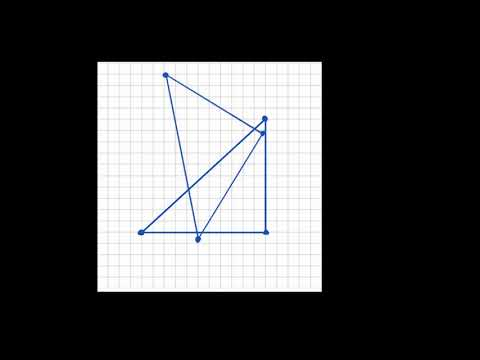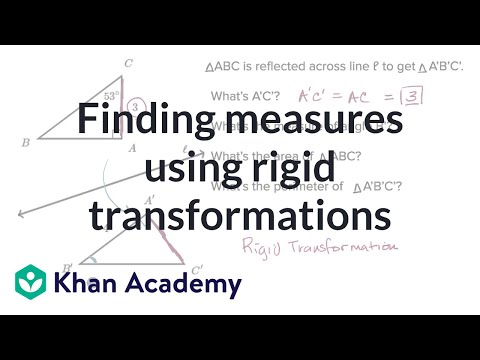COVID-19 presents an unprecedented challenge for teachers, students, and parents. Explore resources from IBM and partners here.

# Determining reflections (Full video)

Description: Finding the line of reflection by considering the image and the source of the reflection. But let's see if we can actually construct a horizontal line where it does actually look like the line of reflection. So let's see, C and C prime, how far apart are they from each other? So let's see if we just put this three above C prime and three below C, let's see if this horizontal line works as a line of reflection.

### Other videos you might be interested in### Dilations intro (Full video)